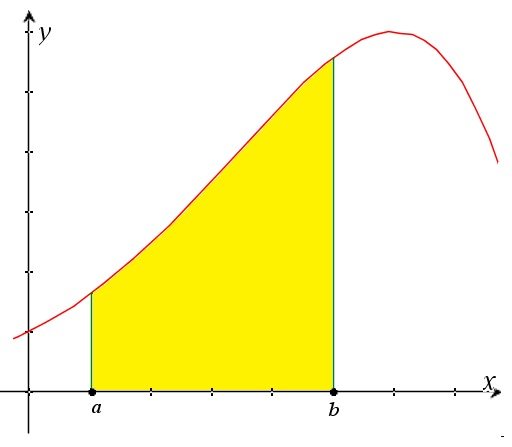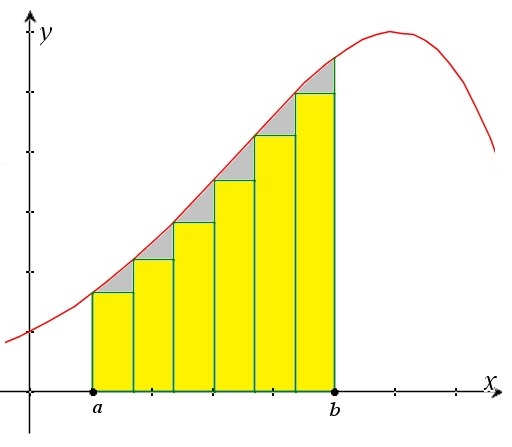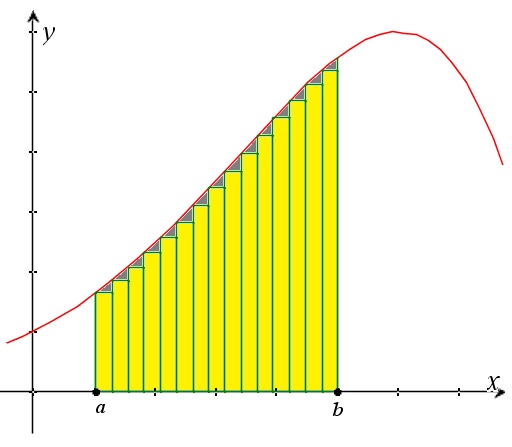# Integrals – a definition – Riemann Integrals

Up a level : Integrals
Next page : The fundamental theorem of calculusIntegrals as areas – Riemann integrals

Assume we have a function y=f (x) and we want to find the area between the function, the x-axis and the lines x=a and x=b. What to do?We could start with approximating the total areas with the help of rectangular areas with the height f (x) and the width Δx for a number of values of x between a and b.  Let us call these values x0=a, x1, x2, and so on  up to xn=b. We will thus have n rectangles where each will have the area ΔA=f (xi) Δx. The sum of these rectangles will approximate the area.We will not get an exact value – the gray areas correspond to the error  – but as we increase the number of rectangles, and thus the width of them the error ought to get smaller and smaller.  We will prove this further down the page.So, assuming the error approaches zero as the number of subdivisions goes toward infinity we have$A = \mathop {\lim }\limits_{n \to \infty} \sum\limits_{i = 0}^{n - 1} {f({x_i})\Delta x}$

where$\Delta x = \frac{{b - a}}{n},\quad {x_i} = a + i\Delta x$

We will now define a definite Riemann integral as$\int\limits_a^b {f(x)dx} = \mathop {\lim }\limits_{n \to \infty} \sum\limits_{i = 0}^{n - 1} {f({x_i})\Delta x}$

The first part reads “the integral from a to b of f of x with respect of x …” The left hand side is just a short form for the left hand side.

Given that the function is positive between a and b and that the limit exist, the integral should give us the area.

Example 1

Let us test this. We will start will a simple example where we know the answer. Let f (x)=x, a=0 and b=2.  We thus get a triangle with the base two and height two, and thus the area 2.

The integral will now be$\begin{gathered} \int\limits_a^b {f(x)dx} = \int\limits_0^2 {xdx} \hfill \\ \quad = \mathop {\lim }\limits_{n \to \infty } \sum\limits_{i = 0}^{n - 1} {x\Delta x = } \mathop {\lim }\limits_{n \to \infty } \sum\limits_{i = 0}^{n - 1} {(a + i\Delta x)\Delta x = } \hfill \\ \quad = \mathop {\lim }\limits_{n \to \infty } \Delta x\sum\limits_{i = 0}^{n - 1} {(a + i\Delta x)} \hfill \\ \end{gathered}$

The sum is an arithmetic series with the first term equal to a and the last equal to bΔx, as you may verify. Since the sum an arithmetic series equals the average of the first and the last term times the number of terms we get

This gives us that$\begin{gathered} \mathop {\lim }\limits_{n \to \infty } \Delta x\sum\limits_{i = 0}^{n - 1} {(a + i\Delta x)} \hfill \\ \quad = \mathop {\lim }\limits_{n \to \infty } \Delta x\frac{{a + b - \Delta x}}{2}n \hfill \\ \quad = \mathop {\lim }\limits_{n \to \infty } \frac{{b - a}}{n}\frac{{a + b - \Delta x}}{2}n \hfill \\ \quad = \mathop {\lim }\limits_{n \to \infty } \frac{1}{2}(b - a)(a + b - \Delta x) \hfill \\ \quad = \mathop {\lim }\limits_{n \to \infty } \frac{1}{2}(b - a)(a + b - \frac{{b - a}}{n}) \hfill \\ \end{gathered}$

Here we used the value for Δx given above. We may now let n go toward infinity to get$\begin{gathered} \mathop {\lim }\limits_{n \to \infty } \Delta x\sum\limits_{i = 0}^{n - 1} {(a + i\Delta x)} \hfill \\ \quad = \mathop {\lim }\limits_{n \to \infty } \frac{1}{2}(b - a)(a + b - \frac{{b - a}}{n}) \hfill \\ \quad = \frac{1}{2}(b - a)(a + b) \hfill \\ \quad = \frac{1}{2}(2 - 0)(0 + 2) = \frac{1}{2} \cdot 2 \cdot 2 = 2 \hfill \\ \end{gathered}$

Phew.

We could have simplified it a bit by realizing that we could let the sum go from i=0 to n, since the area of the added rectangle would get to 0 as the width goes to 0. Anyhow, to start with I think we will let do with one example. Secondly, there must be another way to do this. And happily it is, as we will see in the next page.

Proof that the limit exists

As we saw on the previous page the error correspond to the sum of the somewhat triangular gray areas. That sum is definitely less than the sum of the rectangular areas that correspond to ΔyΔx.  I.e.$\sum\limits_{i = 0}^{n - 1} {\Delta y\Delta x} = \Delta x\sum\limits_{i = 0}^{n - 1} {\Delta y}$

But the sum of all Δy´s is simply f (xn-1)-f (x0), and given that both of these are finite, then the limit of a constant times Δx as n goes toward infinity, and thus Δx goes toward 0, is indeed 0, and the error will thus go toward 0. We will look at cases where the function is not finite later on.Up a level : Integrals
Next page : The fundamental theorem of calculusLast modified: Jun 18, 2018 @ 12:37